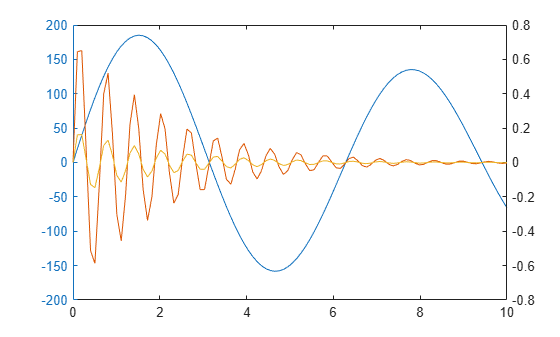# plotyy

(Not recommended) Create graph with two y-axes

`plotyy` is not recommended. Use `yyaxis` instead. For more information on updating your code, see Version History.

## Syntax

``plotyy(X1,Y1,X2,Y2)``
``plotyy(X1,Y1,X2,Y2,f)``
``plotyy(X1,Y1,X2,Y2,f1,f2)``
``plotyy(ax,___)``
``[a,p1,p2] = plotyy(___)``

## Description

example

````plotyy(X1,Y1,X2,Y2)` plots `Y1` against `X1` with y-axis labeling on the left and plots `Y2` against `X2` with y-axis labeling on the right.```
````plotyy(X1,Y1,X2,Y2,f)` uses the specified plotting function to plot the data. ```

example

````plotyy(X1,Y1,X2,Y2,f1,f2)` uses the plotting function `f1` to plot `Y1` against `X1` and the plotting function `f2` to plot `Y2` against `X2`.```
````plotyy(ax,___)` displays the left plot in the specified axes instead of the current axes. If `ax` is a vector, then `plotyy` uses the first axes object in the vector. Specify the axes as the first argument in any of the previous syntaxes.```

example

````[a,p1,p2] = plotyy(___)` returns the two axes objects in `a` and the two graphics objects in `p1` and `p2`. `a(1)` is the left axes and `a(2)` is the right axes. Use `a`, `p1`, and `p2` to modify the properties of the axes and plots after creating them.```

## Examples

collapse all

Plot two data sets on one graph using two y-axes.

```x = 0:0.01:20; y1 = 200*exp(-0.05*x).*sin(x); y2 = 0.8*exp(-0.5*x).*sin(10*x); figure plotyy(x,y1,x,y2)```Plot two data sets using a graph with two y-axes. Add a title and axis labels.

```x = 0:0.01:20; y1 = 200*exp(-0.05*x).*sin(x); y2 = 0.8*exp(-0.5*x).*sin(10*x); figure [hAx,hLine1,hLine2] = plotyy(x,y1,x,y2); title("Multiple Decay Rates") xlabel("Time (\musec)") ylabel(hAx(1),"Slow Decay") % left y-axis ylabel(hAx(2),"Fast Decay") % right y-axis```Plot two data sets using a graph with two y-axes. Change the line styles.

```x = 0:0.01:20; y1 = 200*exp(-0.05*x).*sin(x); y2 = 0.8*exp(-0.5*x).*sin(10*x); [hAx,hLine1,hLine2] = plotyy(x,y1,x,y2); hLine1.LineStyle = "--"; hLine2.LineStyle = ":";```Plot two data sets using a graph with two y-axes. Use a line plot for the data associated with the left y-axes. Use a stem plot for the data associated with the right y-axes.

```x = 0:0.1:10; y1 = 200*exp(-0.05*x).*sin(x); y2 = 0.8*exp(-0.5*x).*sin(10*x); figure plotyy(x,y1,x,y2,@plot,@stem)```Plot three data sets using a graph with two y-axes. Plot one set of data associated with the left y-axis. Plot two sets of data associated with the right y-axis by using two-column matrices.

```x = linspace(0,10); y1 = 200*exp(-0.05*x).*sin(x); y2 = 0.8*exp(-0.5*x).*sin(10*x); y3 = 0.2*exp(-0.5*x).*sin(10*x); figure [hAx,hLine1,hLine2] = plotyy(x,y1,[x',x'],[y2',y3']);```## Input Arguments

collapse all

x-coordinates, specified as a scalar, vector, or matrix.

Data Types: `single` | `double` | `int8` | `int16` | `int32` | `int64` | `uint8` | `uint16` | `uint32` | `uint64` | `categorical` | `datetime` | `duration`

y-coordinates, specified as a scalar, vector, or matrix.

Data Types: `single` | `double` | `int8` | `int16` | `int32` | `int64` | `uint8` | `uint16` | `uint32` | `uint64` | `categorical` | `datetime` | `duration`

Plotting function used to plot the data, specified as a function handle or a character vector that is the name of a plotting function, for example, `semilogx`, `semilogy`, `loglog`, `stem`, or any MATLAB® function that accepts the syntax ```h = function(x,y)```.

Example: `plotyy(x1,y1,x2,y2,@loglog)`

Example: `plotyy(x1,y1,x2,y2,'loglog')`

Target axes, specified as an `Axes` object. If you do not specify the axes, MATLAB plots the first set of data into the current axes or creates an `Axes` object if one does not exist.

## Output Arguments

collapse all

Two axes, returned as an array of two `Axes` objects. `a(1)` is the left axes and `a(2)` is the right axes. Use `a` to modify the properties of the axes.

Graphics object. Use `p` to modify the properties of the plot after creating it.

## Version History

Introduced before R2006a

collapse all

### R2016a: Not recommended

`plotyy` is not recommended. Use `yyaxis` instead. There are no plans to remove `plotyy`.

Starting in R2016a, use the `yyaxis` function to create charts with two y-axes. The `yyaxis` function has several advantages over the `plotyy` function.

• Unlike `plotyy`, the `yyaxis` function creates one `Axes` object with two y-axes. `plotyy` creates two overlaid `Axes` objects that can get out of sync.

• You can use `yyaxis` with any 2-D plotting function. `plotyy` is limited to working with plotting functions of the form `function(x,y)`. It does not work with other plotting functions, such as `errorbar`.

This table shows some typical usages of `plotyy` and how to update your code to use `yyaxis` instead.

Not RecommendedRecommended
`plotyy(x1,y1,x2,y2)`
```yyaxis left plot(x1,y1) yyaxis right plot(x2,y2)```
```plotyy(x1,y1,x2,y2, ... 'function1','function2')```
```yyaxis left function1(x1,y1) yyaxis right function2(x2,y2)```